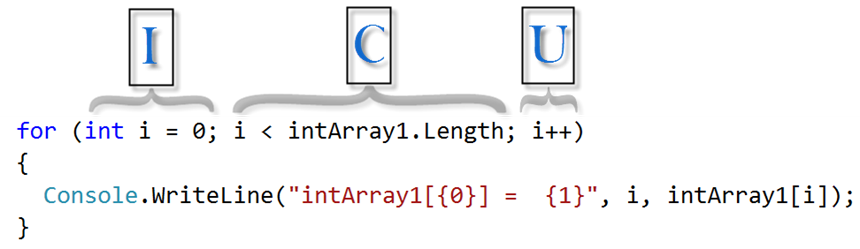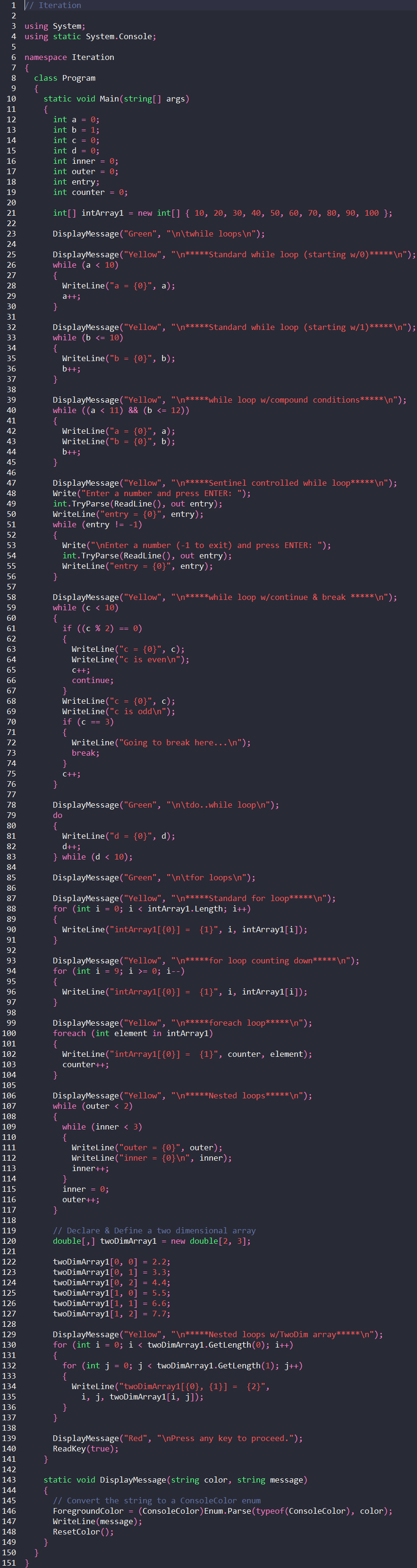# ITSE 1330 | C## Iteration

## Foreword

Recall that the three fundamental programming constructs are sequence, selection, and repetition. Thus far, we have covered sequence and selection. In this chapter, we will work with iteration (repetition). Iteration provides the capability to repeat sections of code until a condition is met. We will consider each of the four iteration alternatives provided by C# which include: while, do..while, for, and foreach. There are many way to implement iteration and we will cover a few variations. We will also review the break and continue statements as they apply to iteration.

## While Loops

The while loop is the most common type of loop. The while and do..while loops do something (execute statements) while a condition is true. That is, the statements in the loop body will be run as long as the condition is true. The conditions are like those we saw in the MakingDecisions chapter. Start a new project named Iteration and code Program.cs as shown. As with previous chapters, when working through the code, compare the code with the output produced.

There are five while loop examples. We will consider three counter-controlled while loops, a sentinel controlled while loop, and a while loop with continue and break statements. While loops are known as pre-test loops because the condition is tested prior to each execution of the loop body. With a pre-test loop, the statements may be executed from 0-to-N times. This means that if the condition is initially not true, the statements will not be executed at all. The do..while loop is a post-test loop and is covered below.

Our first counter-controlled loop begins on line 26. Lines 26-30 contain the simplest form of the while loop and per line 26, will execute (loop) while a < 10. Since the variable "a" starts at 0 (line 12) and is incremented by 1 each time through the loop, the loop statements (lines 28-29) will be run 10 times (0-9). The loop on lines 33-37 is similar to the previous version. The only appreciable differences are that b starts with 1 and the condition is b <= 10 and the output is 1-10.

It is important to note that the number of execution steps for both of the previous loops can be determined by looking at the number in the condition (10 in both cases). In both loops the variable is incremented by 1 each time through the loop. Since "a" starts with 0 and the condition is a < 10, the loop will be executed 10 times. In the next loop, since "b" starts with 1 and the condition is b <= 10, that loop will also be executed 10 times. So, when starting with 0, use <. And, when starting with 1, use <=.

When writing loops, it is critical to include exit criteria that can be satisfied. Otherwise, an infinite loop can be introduced which will never exit. For instance, if line 29 were removed or commented, "a" will always be < 10 and therefore the loop will never exit.

The next loop starting on line 40 uses a compound condition. That loop will be executed as long as both conditions are true. That is, as long as a < 11 and b <= 12. The variable "a" remains 10 in the loop so "a" will always be < 11. However, "b" is incremented by 1 each time through and will reach 13 and therefore the condition b <= 12 becomes false. Note that the logical OR operator (||) can also be used in conditions. If an || were used on line 40, it would be an infinite loop because a < 11 would always be true. Recall that with a logical OR, only one of the conditions need be true.

The next loop starting on line 51 is controlled by a sentinel, or signal. In this case, as long as the variable entry is not equal to -1 the loop will run. There is a ReadLine within a TryParse() method on line 49 in which input is collected from the command line user. This is known as a priming read since the input read is used to prime (start) the loop. The same read statement is contained within the body of the loop on line 54 which is used to keep the loop going. I have not seen a name applied to this common read statement in computer science literature within the loop that corresponds to a priming read. So, let's refer to it as the continuation read since it is used to continue the loop. The sentinel value of -1 is entered by the user when s/he wishes to exit the loop. Note that it is essential to inform the user of the exit criteria which is done on line 53 with the -1 to exit information.

The last while loop we consider is controlled by a counter like some examples discussed above but also includes the continue and break key words. The condition on line 61 is asking if the variable "c" is even which will be the case if the remainder when dividing by 2 == 0. If "c" is even, "c" is incremented and the continue statement is executed. The continue statement on line 66 directs the loop to continue from the top. So, when line 66 executes, program control flow jumps back to line 59 (the top of the loop).

The next time through the loop "c" will not be even so the block from lines 62-67 is skipped. Line 70 asks if c == 3? If so, the condition is satisfied, the message Going to break here... is output and the break statement is executed. Recall from the MakingDecision chapter that the break statement was used to force an exit from the switch control. The break on line 73 is contained within a while statement and will force an exit from the while control when encountered. Like all examples, it is helpful to follow the code results in the output. Furthermore, you should code and modify the examples to increase understanding of the concepts.

## Do..While Loops

Recall that the while loop is a pre-test loop in which the condition is tested before the first run. Our next loop type, the do..while, is a post-test loop in which the loop body is executed once before the first condition test. Lines 79-83 contain a do..while loop. Notice that lines 81 and 82 will be executed before the condition is tested on line 83. The statements in a post-test loop body will be executed 1-toN times.

## For Loops

The for loop is useful when the intent is to process the loop body a specific number of times. Line 88 shows the first for loop example. A considerable advantage of the for loop is that all three loop components (initialization, condition, updated) are contained on one line. The three sections of the for loop are depicted below. In the "I" section, the loop counter is initialized. In the "C" section, the condition is tested, and in the "U" section, an update is performed. The variable "i" is local to the for loop. Initialization is performed once, then the condition test, then the loop statements, then the update, followed by the condition test again.The numbers below show the loop execution sequence. 1, 2, 3, 4, 2, 3, 4, 2, 3, etc. Initialization -> Condition Test -> Loop Body -> Update -> Condition Test -> Loop Body -> etc. Note on line 88 and in the graphic below that the Length property of the array is used to supply the upper boundary of the loop. Also, on line 90, each element of the array is accessed by the loop counter "i". The counter "i" is used to increment the array index.The for loop on lines 94-97 is similar to the previous version but the update counts down instead of up. Notice the >= in the condition test. Also, compare the output of this and the previous loop. What is different and why?

## Foreach Loops

The foreach loop provides a convenient shorthand when working with arrays or other collection types. In the foreach loop on line 100, the local variable "element" is declared within the loop header and is used to store each element of the intArray1[] array. Notice on line 102 that the counter and element are output. We can see in the output that element is equal to each of the array elements in array intArray1[]. So, element is used on line 102 and intArray1[i] is used on line 90 to output the same array contents.

## Nested Loops

An example of nested loops is shown on lines 107-116. The outer loop is executed two times. For each execution of the outer loop, the inner loop is executed three times. To determine the number of times the inner statements of nested loops will be executed, compute the product of the outer times the inner. Therefore, the statements will be run 2 x 3 = 6 times. Compare the output to the nested loop code.

In the next (and last) loop example, we use nested loops to work through the contents of a two-dimensional array. On lines 120-127 a two-dimensional array is created and initialized. We have the nested loops on lines 130-137. Since twoDimArray1[] is a two dimensional array, we use the GetLength() method to determine the length of each dimension. GetLength(0) returns the length of the first dimension (number of rows) and GetLength(1) returns the length of the second dimension (number of columns). Compare the output to the nested loop code.

## Iteration Program.cs## Output Iteration## What's Next?

In the next chapter, we look more closely at the essential elements of object orientation: classes and objects.